Test: Electronic Devices - 2

# Test: Electronic Devices - 2

Test Description

## 20 Questions MCQ Test GATE ECE (Electronics) 2023 Mock Test Series | Test: Electronic Devices - 2

Test: Electronic Devices - 2 for Electronics and Communication Engineering (ECE) 2023 is part of GATE ECE (Electronics) 2023 Mock Test Series preparation. The Test: Electronic Devices - 2 questions and answers have been prepared according to the Electronics and Communication Engineering (ECE) exam syllabus.The Test: Electronic Devices - 2 MCQs are made for Electronics and Communication Engineering (ECE) 2023 Exam. Find important definitions, questions, notes, meanings, examples, exercises, MCQs and online tests for Test: Electronic Devices - 2 below.
Solutions of Test: Electronic Devices - 2 questions in English are available as part of our GATE ECE (Electronics) 2023 Mock Test Series for Electronics and Communication Engineering (ECE) & Test: Electronic Devices - 2 solutions in Hindi for GATE ECE (Electronics) 2023 Mock Test Series course. Download more important topics, notes, lectures and mock test series for Electronics and Communication Engineering (ECE) Exam by signing up for free. Attempt Test: Electronic Devices - 2 | 20 questions in 60 minutes | Mock test for Electronics and Communication Engineering (ECE) preparation | Free important questions MCQ to study GATE ECE (Electronics) 2023 Mock Test Series for Electronics and Communication Engineering (ECE) Exam | Download free PDF with solutions
 1 Crore+ students have signed up on EduRev. Have you?
Test: Electronic Devices - 2 - Question 1

### For a P-channel Ge JFET, it is having doping concentration of Na = 1.77 × 1015 cm−3 and Nd = 3.53 × 1018 per cm3, If channel thickness is a = 1 µ met. (Given Relative permittivity of Ge = 16 at T = 300° K)  Q. What is value of internal pinch-off voltage?

Detailed Solution for Test: Electronic Devices - 2 - Question 1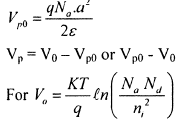Test: Electronic Devices - 2 - Question 2

### For a P-channel Ge JFET, it is having doping concentration of Na = 1.77 × 1015 cm−3 and Nd = 3.53 × 1018 per cm3, If channel thickness is a = 1 µ met. (Given Relative permittivity of Ge = 16 at T = 300° K)  Q. What is value of pinch-off voltage?

Detailed Solution for Test: Electronic Devices - 2 - Question 2Test: Electronic Devices - 2 - Question 3

### If saturation currents of 2 diodes are 1 µA and 2 µA. If break down voltages of diode are same and are equal to 100 volt, what is value of current in D1.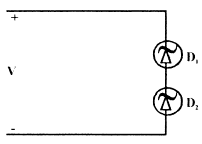Q. If V = 90 volt

Test: Electronic Devices - 2 - Question 4

If saturation currents of 2 diodes are 1 µA and 2 µA.
If break down voltages of diode are same and are equal to 100 volt, what is value of current in D1.Q.

If V = 110 volt

Test: Electronic Devices - 2 - Question 5

For Si transistor, if β ≥ 30 and ICBO = 10 nA  The minimum value of R, for transistor to remain in active region for vi = 12 volt, is __________ kΩ.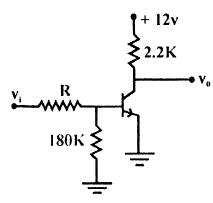Test: Electronic Devices - 2 - Question 6

A sample of silicon (uniformly doped n-type) at T = 300° K has the electron concentration varying linearly with distance as shown in the figure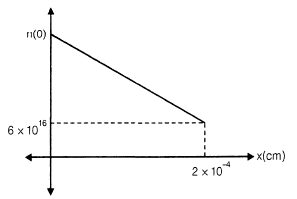The diffusion current is found to be −1120 A/cm2. If the diffusion constant Dn = 35 cm2/s, the electron concentration at x = 0 is

Detailed Solution for Test: Electronic Devices - 2 - Question 6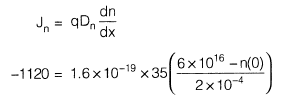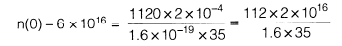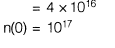Test: Electronic Devices - 2 - Question 7

In a semiconductor sample,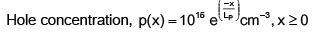where LP (hole diffusion length) = 4.8 × 10−4 cm

Hole diffusion coefficient DP = 20 cm2/s

The hole diffusion current density at x = 0 is

Detailed Solution for Test: Electronic Devices - 2 - Question 7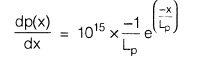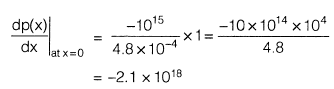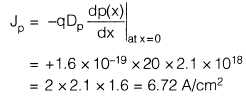Test: Electronic Devices - 2 - Question 8

In the plot of log I vs V for a semiconductor Ge diode, the slope at room temperature is _________. Assume V >> VT and room temperature 27°C.

Detailed Solution for Test: Electronic Devices - 2 - Question 8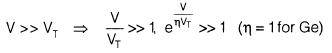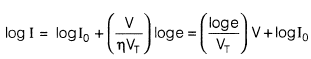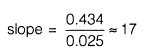Test: Electronic Devices - 2 - Question 9

Consider a p-type semiconductor which is lightly doped i.e. condition of p >> n is not valid. p, n, ni are holes, electrons and intrinsic carrier concentration respectively. Then 2n + NA is ___________ if NA is immobile acceptor ions concentration

Detailed Solution for Test: Electronic Devices - 2 - Question 9

According to charge neutrality,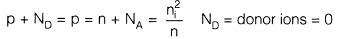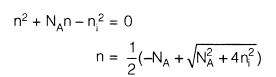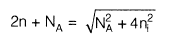Test: Electronic Devices - 2 - Question 10

Consider the following network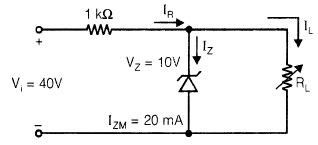VL is to be maintained at 10 V.
The correct representation of VL versus RL is

Detailed Solution for Test: Electronic Devices - 2 - Question 10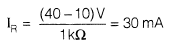RLmin to turn-on the zener diode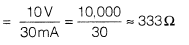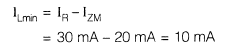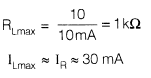Test: Electronic Devices - 2 - Question 11

Consider the following sentences in respect of LEDs (Light emitting diodes) and semiconductor laser diode.
S1 :  Only direct band gap type semiconductors are suitable for fabrication of LEDs.
S2 : Both direct band gap type and indirect band gap type semiconductors are suitable for fabrication of semiconductor laser diode.
Choose the best alternative

Detailed Solution for Test: Electronic Devices - 2 - Question 11

For the fabrication of semiconductor laser diode, direct band gap type semiconductor is required

Test: Electronic Devices - 2 - Question 12

If individual JFET has IDSS = 5 mA and pinch-off voltage  VP0 = 3V ,  then current iD for VGS = − 0.9 V is __________ mA.

Detailed Solution for Test: Electronic Devices - 2 - Question 12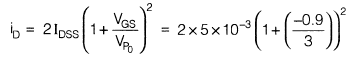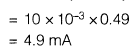Test: Electronic Devices - 2 - Question 13

What are the states of three ideal diodes of circuit shown below: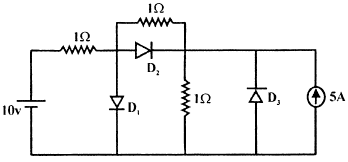Test: Electronic Devices - 2 - Question 14

For BJT, circuit shown assume that β of transistor is very large, if here Si transistor is used, then transistor will operate in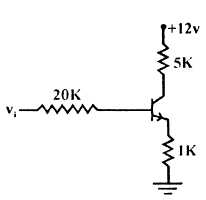Detailed Solution for Test: Electronic Devices - 2 - Question 14

If β is large then transistor will be in S.R

Test: Electronic Devices - 2 - Question 15

Consider the following circuit: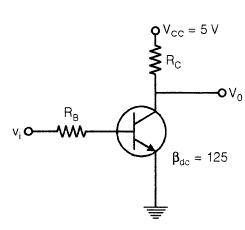Q.

If vi is as shown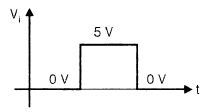then RB and RC for the circuit concerned can be _________ and _________ respectively, if ICsat = 6 mA. Assume the transistor inverter operation

Detailed Solution for Test: Electronic Devices - 2 - Question 15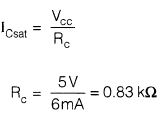Just at onset of saturation,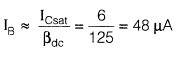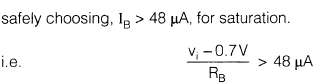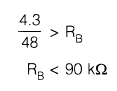Test: Electronic Devices - 2 - Question 16

Consider the following circuit:Q.

The V0 shall be represented by

Detailed Solution for Test: Electronic Devices - 2 - Question 16

Transistor is switch in inverter operation.

Test: Electronic Devices - 2 - Question 17

A BJT having β = 125 is biased at a dc collector current of 1.23 mA. The values of gm, re and rπ at the bias point are: (Assume temperature of operation = 25°C).

Detailed Solution for Test: Electronic Devices - 2 - Question 17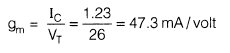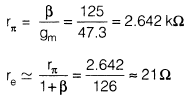Test: Electronic Devices - 2 - Question 18

A properly biased JFET may be shown by one of the following figures.Choose the correct alternative: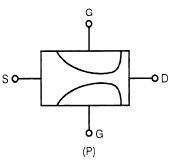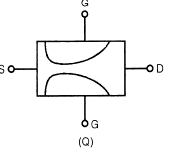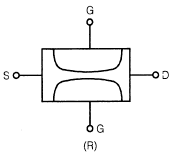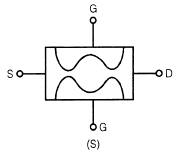Detailed Solution for Test: Electronic Devices - 2 - Question 18

Because of the more reverse biased drain end, the depletion width is thinner in drain side.

Test: Electronic Devices - 2 - Question 19

Shown below is the n-channel JFET in voltage divider bias arrangement.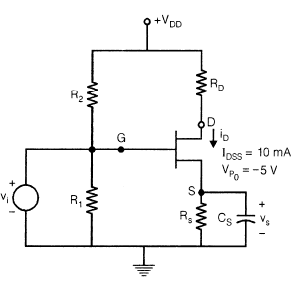VDD = 12 V
RS = RD = 1.8 kΩ

R1 = 100 kΩ

R2 = 400 kΩ

Q.

Gate to source voltage (VGS) is given by the quadratic equation

V2GS + PVGS + Q = 0
P and Q are respectively

Detailed Solution for Test: Electronic Devices - 2 - Question 19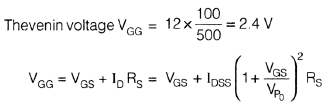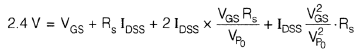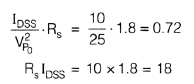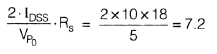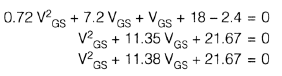Test: Electronic Devices - 2 - Question 20

Shown below is the n-channel JFET in voltage divider bias arrangement.VDD = 12 V
RS = RD = 1.8 kΩ

R1 = 100 kΩ

R2 = 400 kΩ

Q.

The drain current iD is

Detailed Solution for Test: Electronic Devices - 2 - Question 20

Solving VGS from the obtained quadratic equation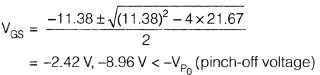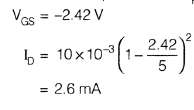## GATE ECE (Electronics) 2023 Mock Test Series

21 docs|263 tests
Information about Test: Electronic Devices - 2 Page
In this test you can find the Exam questions for Test: Electronic Devices - 2 solved & explained in the simplest way possible. Besides giving Questions and answers for Test: Electronic Devices - 2, EduRev gives you an ample number of Online tests for practice

## GATE ECE (Electronics) 2023 Mock Test Series

21 docs|263 tests(Scan QR code)